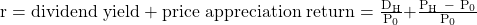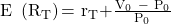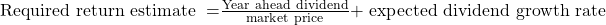Essential Concept 43: Return Concepts | IFT World
101 Concepts for the Level I Exam

# Essential Concept 43: Return ConceptsHolding period return is the return earned from investing in an asset over a specified period of time.Realized return is the holding period return earned in the past. Expected return is the expected holding-period return for a stock in the future based on expected dividend yield and the expected price appreciation return.

Required return is the minimum level of expected return that an investor requires over a specified period of time, given the asset’s riskiness.

Asset’s expected alpha or expected abnormal return is the difference between the expected return and the required rate of return on an asset. It is given by the formula:

Expected alpha (ex-ante alpha) = Expected return – Required return

Realized alpha = Actual holding-period return – Contemporaneous required return

Contemporaneous return is the return on other investments with similar risk profile during the same holding period.

When an investor’s estimate of intrinsic value of an asset differs from its market price, the asset is mispriced. For a mispriced asset, the investor expects to earn the required return plus the return from the convergence of price to intrinsic value.The discount rate is any rate used in finding the present value of a future cash flow. Market required return is often used as a discount rate.

The internal rate of return (IRR) is the discount rate that equates the present value of the asset’s expected future cash flows to the asset’s price. If an asset is correctly priced (market price = intrinsic value), then IRR = required return.** IFT Website Un-available on Monday 2-October-2023, 0700-1200 GMT **
This is default text for notification bar